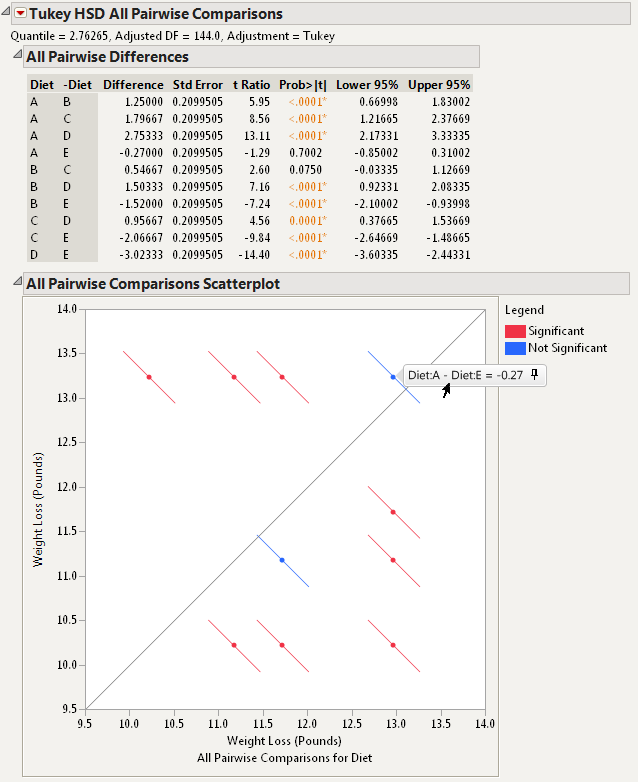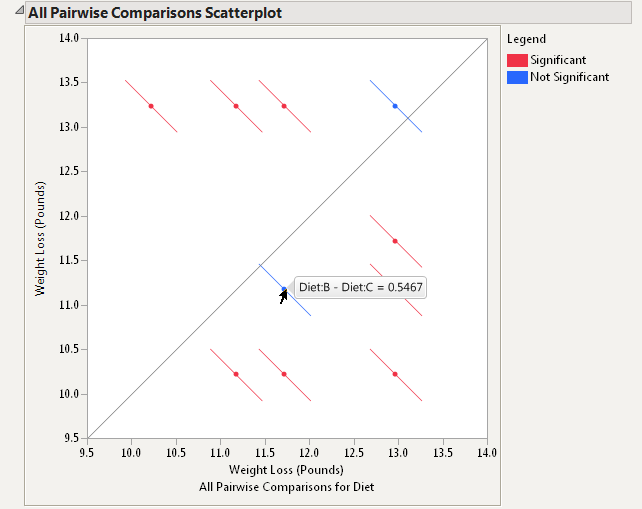Our World Statistics Day conversations have been a great reminder of how much statistics can inform our lives. Do you have an example of how statistics has made a difference in your life? Share your story with the Community!
Choose Language Hide Translation Bar
More Multiple Comparisons options in JMP 11: All Pairwise Comparisons

In my last blog post, I introduced the new Multiple Comparisons option available in Fit Model Least Squares in JMP 11 and showed an example where we compared the treatment means of two effects to the overall average. We learned how to examine a Comparison with Overall Average Decision Chart, also known as an ANOM chart, to determine which treatment means were significantly different from the overall average and to assess practical significance. This blog post will demonstrate the use of Multiple Comparisons to perform all pairwise comparisons of treatment means.

We will look at data from an experiment that was conducted to evaluate the effectiveness of five different diets on men and women. Each of the five diets was assigned to 15 men and 15 women who were at least 50 pounds overweight. The subjects followed their assigned diet for four weeks, and their weight loss was recorded at the end of the four weeks.

The researchers fit a least squares model to the weight loss data with the main effects of the two factors, diet and gender. Now the researchers would like to compare the mean weight loss of all pairs of diets to see which diets are significantly different from each other. Once again, we can answer this question using “Multiple Comparisons” under the “Estimates” submenu.  Figure 1 shows the Multiple Comparisons launch dialog with the default estimate type, “Least Squares Means Estimates,” the effect, “Diet,” selected, and the Comparison, “All Pairwise Comparisons – Tukey HSD,” checked.

The Tukey HSD All Pairwise Comparisons report is shown below the least squares means estimates in the Multiple Comparisons Report and is displayed in Figure 2. All of the pairwise differences of the least squares means estimates are shown in both a table and graphically in the All Pairwise Comparisons Scatterplot, also sometimes called a diffogram or mean-mean scatterplot. (Note: We chose the Tukey HSD pairwise comparisons instead of Student’s t because it is constructed so that the significance level applies jointly to all of the pairwise comparisons.)In an All Pairwise Comparisons Scatterplot, the points are plotted at the coordinates of each pair of least squares means estimates. The diagonal line represents the place where all of the least squares means would be equal. You can see the label for each point by hovering the cursor over the point. This is shown in Figure 2 for the point that compares Diet A and Diet E. (Note: It is possible to make the label persist on the graph by clicking on the pin on the right side of the label.) The line segments correspond to the confidence intervals for each pairwise difference. If the line segment crosses the diagonal, the pair being compared is not statistically significantly different and is color-coded blue. If the line segment does not cross the diagonal, the pair being compared is statistically significantly different and is color-coded red.

In the All Pairwise Comparisons Scatterplot in Figure 2, we see that only two line segments cross the diagonal. Thus, all pairs of diets are significantly different from each other except for the two pairs with line segments that cross the diagonal. One of the pairs of diets that is not significantly different is Diet A and Diet E, as seen by the label shown in Figure 2. The difference in the least squares means of these two diets is just 0.27 pounds. By hovering over the other blue point in the graph, as illustrated in Figure 3, we see that Diets B and C are also not significantly different. The difference in the least squares means of these two diets is 0.5467 pounds. The rest of the pairs are significantly different, and the farther the pair is from the diagonal line the bigger the difference between the pair.In my next blog post, we will continue looking at all pairwise comparisons, but we will answer a different question. Instead of trying to determine which pairs of treatments means are significantly different, we will try to determine if any pairs are practically equivalent.

Article Labels

There are no labels assigned to this post.

Article Tags